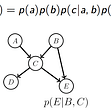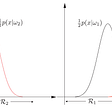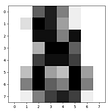# Machine Learning Algorithms In A Whole.

1. Linear Regression
• 3.1 − Calculate the distance between test data and each row of training data with the help of any of the method namely: Euclidean, Manhattan or Hamming distance. The most commonly used method to calculate distance is Euclidean.
• 3.2 − Now, based on the distance value, sort them in ascending order.
• 3.3 − Next, it will choose the top K rows from the sorted array.
• 3.4 − Now, it will assign a class to the test point based on most frequent class of these rows.
• Decision tree algorithm falls under the category of supervised learning. They can be used to solve both regression and classification problems.
• Decision tree uses the tree representation to solve the problem in which each leaf node corresponds to a class label and attributes are represented on the internal node of the tree.
• We can represent any boolean function on discrete attributes using the decision tree.
• Step-1: Begin the tree with the root node, says S, which contains the complete dataset.
• Step-2: Find the best attribute in the dataset using Attribute Selection Measure (ASM).
• Step-3: Divide the S into subsets that contains possible values for the best attributes.
• Step-4: Generate the decision tree node, which contains the best attribute.
• Step-5: Recursively make new decision trees using the subsets of the dataset created in step -3. Continue this process until a stage is reached where you cannot further classify the nodes and called the final node as a leaf node.

## Attribute Selection Measures

1. Information Gain
2. Gini Index
• It calculates how much information a feature provides us about a class.
• According to the value of information gain, we split the node and build the decision tree.
• A decision tree algorithm always tries to maximize the value of information gain, and a node/attribute having the highest information gain is split first.
`Entropy(s)= -P(yes)log2 P(yes)- P(no) log2 P(no)`
• S= Total number of samples
• P(yes)= probability of yes
• P(no)= probability of no
• An attribute with the low Gini index should be preferred as compared to the high Gini index.
• It only creates binary splits, and the CART algorithm uses the Gini index to create binary splits.
• Gini index can be calculated using the below formula:
`Gini Index= 1- ∑jPj2`
1. The random forest algorithm is not biased, since, there are multiple trees and each tree is trained on a subset of data. Basically, the random forest algorithm relies on the power of “the crowd”; therefore the overall biasedness of the algorithm is reduced.
2. This algorithm is very stable. Even if a new data point is introduced in the dataset the overall algorithm is not affected much since new data may impact one tree, but it is very hard for it to impact all the trees.
3. The random forest algorithm works well when you have both categorical and numerical features.
4. The random forest algorithm also works well when data has missing values or it has not been scaled well (although we have performed feature scaling in this article just for the purpose of demonstration).
1. A major disadvantage of random forests lies in their complexity. They required much more computational resources, owing to the large number of decision trees joined together.
2. Due to their complexity, they require much more time to train than other comparable algorithms.

# Pros & Cons of Support Vector Machines

• Accuracy
• Works well on smaller cleaner datasets
• It can be more efficient because it uses a subset of training points
• Isn’t suited to larger datasets as the training time with SVMs can be high
• Less effective on noisier datasets with overlapping classes

# SVM Uses

• Naïve Bayes algorithm is a supervised learning algorithm, which is based on Bayes theorem and used for solving classification problems.
• It is mainly used in text classification that includes a high-dimensional training dataset.
• Naïve Bayes Classifier is one of the simple and most effective Classification algorithms which helps in building the fast machine learning models that can make quick predictions.
• It is a probabilistic classifier, which means it predicts on the basis of the probability of an object.
• Some popular examples of Naïve Bayes Algorithm are spam filtration, Sentimental analysis, and classifying articles

# Advantages of Naïve Bayes Classifier:

• Naïve Bayes is one of the fast and easy ML algorithms to predict a class of datasets.
• It can be used for Binary as well as Multi-class Classifications.
• It performs well in Multi-class predictions as compared to the other Algorithms.
• It is the most popular choice for text classification problems.

# Disadvantages of Naïve Bayes Classifier:

• Naive Bayes assumes that all features are independent or unrelated, so it cannot learn the relationship between features.

# Applications of Naïve Bayes Classifier:

• It is used for Credit Scoring.
• It is used in medical data classification.
• It can be used in real-time predictions because Naïve Bayes Classifier is an eager learner.
• It is used in Text classification such as Spam filtering and Sentiment analysis.

# What is K-Means Algorithm?

• Determines the best value for K center points or centroids by an iterative process.
• Assigns each data point to its closest k-center. Those data points which are near to the particular k-center, create a cluster.

# How To Train One Model

--

--

--

## More from HITARTH SHAH

Data Science, ML, DL,AI, Researcher

Love podcasts or audiobooks? Learn on the go with our new app.

## Recommended from Medium## Building Your Own AI Stand-Up Comedian## Classification A tour of the Classics: Zero_ML## Support Vector Machine — Basics## Implementing The Perceptron Algorithm From Scratch In Python## Cat or Dog? Introduction to Naive Bayes## Albumentations: A Python library for advanced Image Augmentation strategies## HITARTH SHAH

Data Science, ML, DL,AI, Researcher

## What is Machine Learning## Allstate Claims Severity — How severe is an insurance claim?## Beginners Guide to Machine learning